Wednesday 17th August 2022
 All India Engineering / Architecture Entrance Examination (AIEEE) CBSE Guess > AIEEE > AIEEE Papers > 2007 > Physics AIEEE 2007 Physics Q. 1. The displacement of an object attached to a spring and executing simple harmonic motion is given by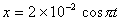metres. The time at which the maximum speed first occurs is 0.5 s 0.75 s 0.125 s 0.25 s Sol: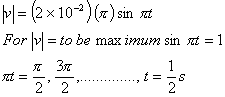Correct choice: (1) Q. 2. In an a.c. circuit the voltage applied is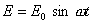. The resulting current in the circuit is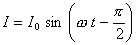. The power consumption in the circuit is given by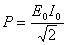P = zero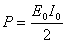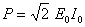Sol.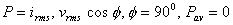Correct choice: (2) Q. 3. An electric charge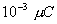is placed at the origin (0, 0) of X – Y co-ordinate system. Two points A and B are situated at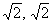and (2, 0) respectively. The potential difference between the points A and B will be 9 volt zero 2 volt 4.5 volt Sol: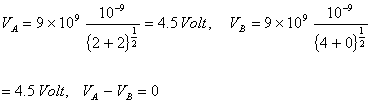Correct choice: (2) Q. 4. A battery is used to charge a parallel plate capacitor till the potential difference between the plates becomes equal to the electromotive force of the battery. The ratio of the energy stored in the capacitor and the work done by the battery will be 1 2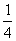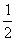Sol: Work done by battery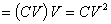Energy stored in capacitor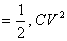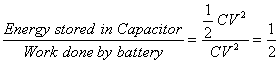Correct choice: (4)